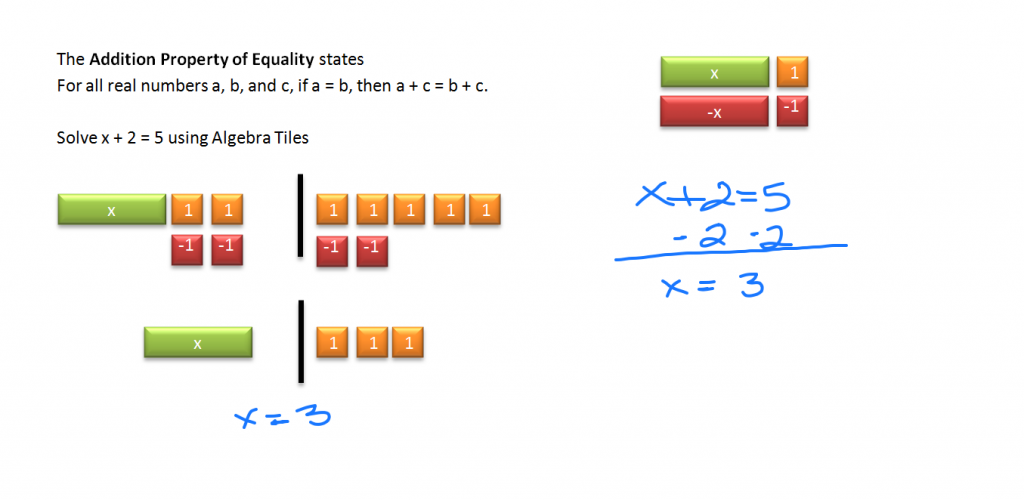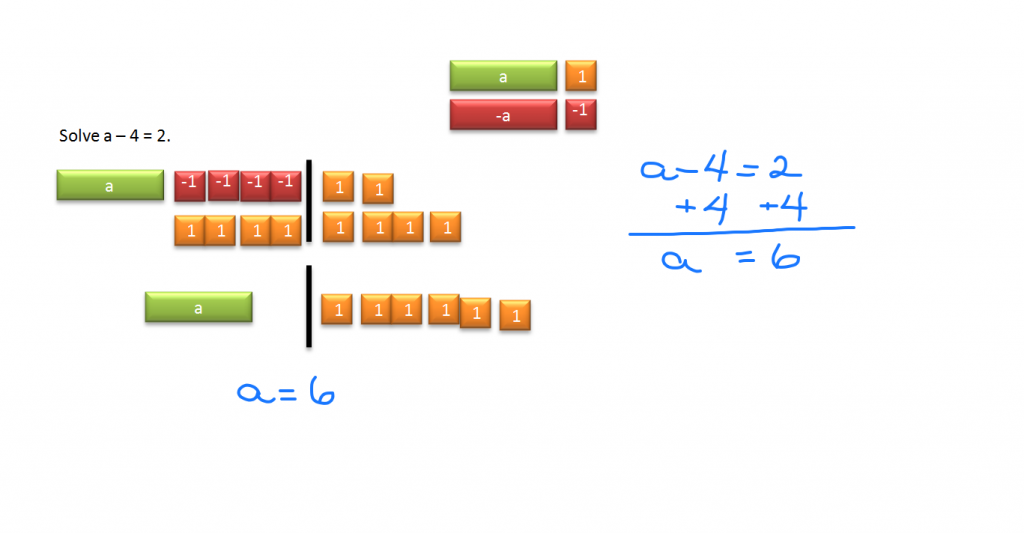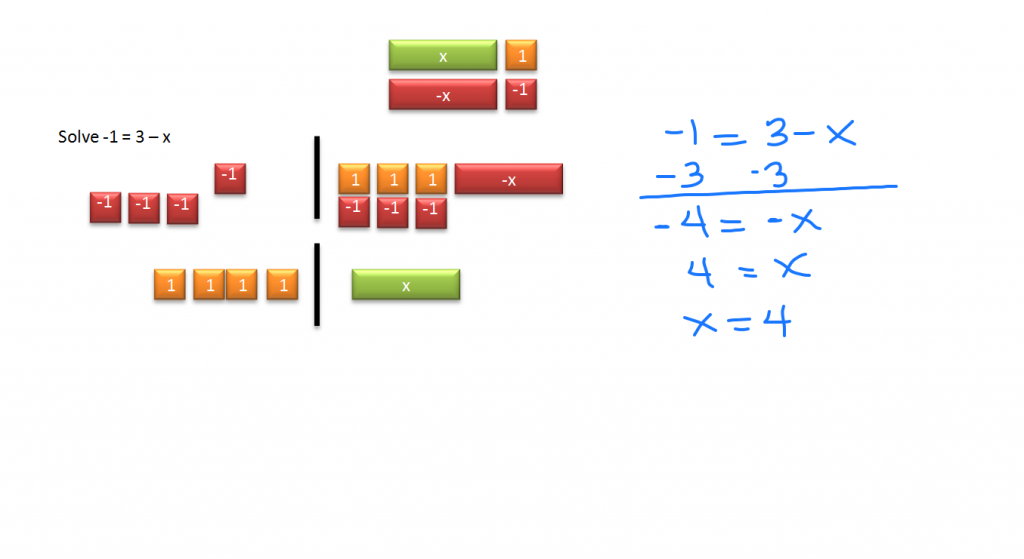# Solve Equations by Adding & Subtracting

//Solve Equations by Adding & Subtracting
Solve Equations by Adding & Subtracting 2017-11-13T22:01:52+00:00

Algebra tiles can help you visualize what’s actually happening when you are solving algebraic equations.

Tips: Be sure the tile itself and not the text is selected when making a copy.

You can:

• Right click and copy
• Select the tile and press Ctrl + D
• Use the Ctrl + C, Ctrl + V copy and paste short cuts or
• Hold the Ctrl key down while dragging a tile which also makes a copy of the original

The Addition Property of Equality states

For all real numbers a, b, and c, if a = b, then a + c = b + c.

Solve x + 2 = 5 using Algebra Tiles

The black bar stands for the equal sign. The first step is to copy the tiles above to model the equation. Once the equation has been formed, the next step is to isolate the variable x. It currently has two unit tiles added to it. The addition property of equality says if we add the same number to both sides of the equation, we have an equivalent equation. To eliminate the two ones tiles on the left side of the equation, we need to add the opposite. Copy two negative ones tiles to the left side, but also do the same to the right side.  The zero pairs are eliminated. And we are left with x = 3.Solve a – 4 = 2.

The great thing about electronic algebra tiles is that you can change the tile name to reflect that this problem is using a instead of x. Now repeat the process above. Copy an a tile and four negative 1 tiles to the left side. Copy two positive one tiles to the right. Add the tiles to the left that will get the variable a by itself, but also add the same number of one tiles to the right side.The result is that a = 6.

Solve -1 = 3 – xA teacher demo file, a student activity document, and the key to the student activity are available for download for use in your classroom or independent learning.

Text:

Holt, Rinehart and Winston Algebra 1 p.114 – 121